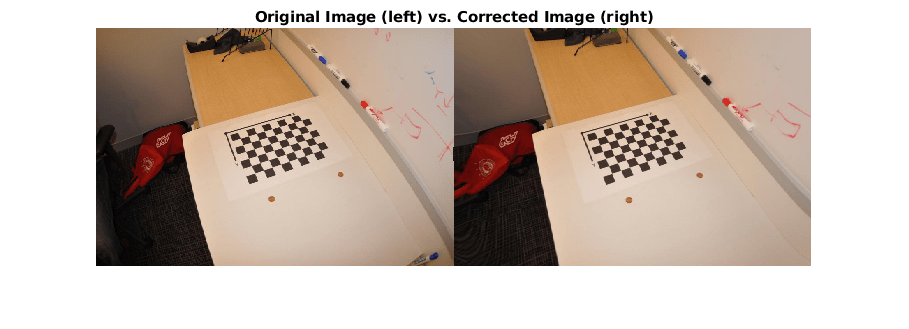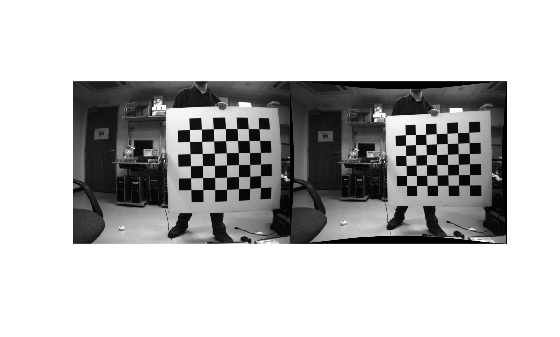# cameraIntrinsicsFromOpenCV

Convert camera intrinsic parameters from OpenCV to MATLAB

## Syntax

``intrinsics = cameraIntrinsicsFromOpenCV(intrinsicMatrix,distortionCoefficients,imageSize)``

## Description

example

````intrinsics = cameraIntrinsicsFromOpenCV(intrinsicMatrix,distortionCoefficients,imageSize)` converts the OpenCV intrinsics, specified by the input arguments, into a MATLAB® `cameraIntrinsics` object `intrinsics`.The OpenCV spatial coordinate system specifies the upper-left pixel center at (`0,0`), whereas the MATLAB spatial coordinate system specifies the pixel center at (`1,1`). The `cameraIntrinsicsFromOpenCV` function compensates for this difference by adding 1 to both of the x and y-values for the converted principal point.OpenCV intrinsics cannot be converted to a MATLAB `cameraIntrinsics` object when: The OpenCV pinhole camera model uses more than five distortion coefficients.An OpenCV fisheye model is used. In these cases, you can recalibrate the stereo camera using the Camera Calibrator app.```

## Examples

collapse all

Define OpenCV camera intrinsic parameters in the workspace.

```intrinsicMatrix = [729.4644 0 570.6455; 0 728.8196 346.0108; 0 0 1 ]; distortionCoefficients = [-0.4262 0.5460 0.0038 -0.0051 -0.6176];```

Define the image size returned by the camera.

`imageSize = [712 1072];`

Convert the intrinsic parameters from OpenCV to MATLAB format.

```intrinsics = cameraIntrinsicsFromOpenCV(intrinsicMatrix, ... distortionCoefficients,imageSize);```

```filename = fullfile(toolboxdir("vision"),"visiondata","calibration", ... "mono","image01.jpg"); I = imread(filename);```

Undistort the image and diplay the results.

```J = undistortImage(I,intrinsics); imshowpair(I,J,"montage"); title("Original Image (left) vs. Corrected Image (right)");```The ROS camera calibration package estimates camera intrinsic parameters using the OpenCV camera calibration tools . After calibrating a camera in ROS, you can import its intrinsic parameters to a YAML file using the camera calibration parser in ROS. To use the calibrated camera with Computer Vision Toolbox™ functions, such as `undistortImage`, you must read the camera parameters from the YAML file and then convert them into a `cameraIntrinsics` object using `cameraIntrinsicsFromOpenCV`.

Note: The `cameraIntrinsicsFromOpenCV` function supports importing camera intrinsic parameters for only those pinhole camera models that use the `ROS plumb-bob` distortion model.

Read Camera Intrinsic Parameters from a ROS YAML File

Read the camera parameters stored in `cameraParams.yaml` using the helper function `helperReadYAML`.

`intrinsicsParams = helperReadYAML('cameraParams.yaml');`

Create `cameraIntrinsics` Object Using `cameraIntrinsicsFromOpenCV`

Use the `cameraIntrinsicsFromOpenCV` function to create a `cameraIntrinsics` object from the camera matrix and the distortion coefficients.

```imageSize = [intrinsicsParams.image_height intrinsicsParams.image_width]; intrinsicMatrix = intrinsicsParams.camera_matrix; distortionCoefficients = intrinsicsParams.distortion_coefficients; intrinsicsObj = cameraIntrinsicsFromOpenCV(intrinsicMatrix,distortionCoefficients,imageSize);```

Undistort Image

Use the imported camera intrinsics with `undistortImage` to undistort an image captured using the calibrated camera.

```% Load the captured image. imageName = fullfile(toolboxdir('vision'),'visiondata','calibration','stereo','left','left01.png'); I = imread(imageName); % Undistort the image. J = undistortImage(I,intrinsicsObj,'OutputView','full'); % Display the result. figure montage({I,J})```Supporting Functions

The `helperReadYAML` function reads the monocular camera parameters from the input YAML file that was exported from ROS.

```function cameraParams = helperReadYAML(filename) % helperReadYAML reads a ROS YAML file, filename, and returns a structure % with these fields: image_width, image_height, camera_name, % camera_matrix, distortion_model, distortion_coefficients, % rectification_matrix, and projection_matrix. These fields are stored % in the YAML file colon separated from their values in different lines. f = fopen(filename,'r'); stringFields = {'camera_name','distortion_model'}; while ~feof(f) [name,value,isEmptyLine] = helperReadYAMLLine(f); if isEmptyLine continue end if ~isempty(value) % Convert all values to numbers except for known string % fields. if ~any(contains(name, stringFields)) value = str2num(value); %#ok end else % An empty value in ROS YAML files indicates a matrix in % upcoming lines. Read the matrix from the upcoming lines. value = helperReadYAMLMatrix(f); end % Store post-processed value. cameraParams.(name) = value; end fclose(f); end```

The `helperReadYAMLMatrix` function reads the rows, columns and data fields of a matrix in the ROS YAML file.

```function matrix = helperReadYAMLMatrix(f) % helperReadYAMLMatrix reads a matrix from the ROS YAML file. A matrix in % a ROS YAML file has three fields: rows, columns and data. rows and col % describe the matrix size. data is a continguous array of the matrix % elements in row-major order. This helper function assumes the presence % of all three fields of a matrix to return the correct matrix. numRows = 0; numCols = 0; data = []; % Read numRows, numCols and matrix data. while ~feof(f) [name,value,isEmptyLine] = helperReadYAMLLine(f); if isEmptyLine continue end switch name case 'rows' numRows = str2num(value); %#ok case 'cols' numCols = str2num(value); %#ok case 'data' data = str2num(value); %#ok % Terminate the while loop as data is the last % field of a matrix in the ROS YAML file. break otherwise % Terminate the while loop if any other field is % encountered. break end end if numel(data) == numRows*numCols % Reshape the matrix using row-major order. matrix = reshape(data,[numCols numRows])'; end end```

The `helperReadYAMLLine` function reads a line of a ROS YAML file.

```function [name,value,isEmptyLine] = helperReadYAMLLine(f) % Read line from file. line = fgetl(f); % Trim leading and trailing whitespaces. line = strtrim(line); if isempty(line) || line(1)=='#' % Empty line or comment. name = ''; value = ''; isEmptyLine = true; else % Split the line to get name and value. c = strsplit(line,':'); assert(length(c)==2,'Unexpected file format') name = c{1}; value = strtrim(c{2}); % Trim leading whitespace. isEmptyLine = false; end end```

References

## Input Arguments

collapse all

Camera intrinsic matrix from OpenCV, specified as a 3-by-3 matrix of the form:

`$\left[\begin{array}{ccc}fx& 0& cx\\ 0& fy& cy\\ 0& 0& 1\end{array}\right]$`

where fx and fy are the focal lengths in the x and y-directions, and (cx,cy) is the principal point in OpenCV.

Camera radial and tangential distortion coefficients from OpenCV, specified as a five-element vector of the form [k1 k2 p1 p2 k3]. The values of k1, k2, and k3 describe the radial distortion and p1 and p2 describe the tangential distortion, specified in OpenCV.

Image size, specified as a 2-element vector in the form [mrows,ncols].

## Output Arguments

collapse all

Camera intrinsic parameters, returned as a `cameraIntrinsics` object.

## Version History

Introduced in R2021b